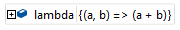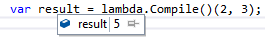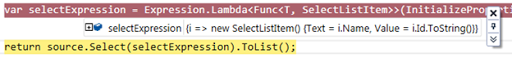### Create Dynamic Linq using Expressions– Basic to Advance

In previous post we learnt basic understanding of Expression and few insights how Expression represents the code as Data. Also we learn how to compile and invoke an Expression.
In this post you’ll learn

- Creating a simple lambda expression

- Createing a dynamic Linq expression

Before moving ahead I want to mention the MSDN documentation of Expression class to go through for its methods and properties available to build code.

Creationg a simple lambda expression

Let’s take a sample expression which adds up two numbers using a delegate.

Expression<Func<int, int, int>> sumExpr = (a, b) => a + b;

This is how we can write it in the code but it’s possible to build such expressions on the fly. The non-generic Expression class provides a lots of methods and properties.

If we parse this expression, (see previous article) we’ll get something like below:

Parameters = {a, b}

Body = a + b

NodeType = Lambda

So we would need to declare two parameters first. Which we can do like below:

`var paramExprA = Expression.Parameter(typeof(int), "a");`
`var paramExprB = Expression.Parameter(typeof(int), "b");`

Now let’s build the body, as we can see the body is a binary operation being performed with add operator. So it will be a binary expression, with Add method and pass those parameters expression to it.

`var body = BinaryExpression.Add(paramExprA, paramExprB);`

So now we have our parameters and body. Node type will stick them together:

`var lambda = Expression.Lambda<Func<int, int, int>>(body, paramExprA, paramExprB);`

We have defined the Lambda type as Func with two argument of int and return type int. Then supply the body and parameters expression. And the expression is complete. Now if you look at the lambda in debug mode it will look like exactly that it supposed to be.Now to execute it, we have to do the same as declared Expressions.

`var result = lambda.Compile()(2, 3);`The method can be generalized with generics and then it can be called with any types:

You can call it like:
var res = BuildIt<int>().Compile()(2, 3);
var res = BuildIt<decimal>().Compile()(2.4, 3.5);
var res = BuildIt<string>().Compile()(“Jones”, “Smith”);

I hope you must be comfortable building the expressions, so let’s move on and build a little complex and useful expression which would help reducing the duplicate code.

Creating a simple dynamic Linq expression

Now we have a class ‘TestDemo’ and we want to convert the list of objects of `TestDemo` to another list of objects of class ‘SelectListItem’. This is a class in Asp.net MVC which represents the items source of HTML Dropdownlist and its very common case. So assuming that we have 10-11 types of such classes like `TestDemo` which would get converted to Dropdownlist to show on view. In real scenario, let’s assume these classes are DbContext entities pulling data from DB and converting them to Dropdownlist compatible objects.

For multiple classes the LINQ would go like:

This code is one of the question from SO. Where the person asked to refactor this code. And ofcourse this looks bit awkward to have same LINQ select criteria repeated to all classes. Now assume if you have such 10-12 classes how the method would look.

So the solution was to create a Generic method which can supply the expression and return the list of ‘SelectListItem’.

Let’s define the signature of the generic method –

`public static IEnumerable<SelectListItem> GetList<T>(this IQueryable<T> source)`

This is a generic Extension method for IQueryables so it can be invoked via any IQueryable type. And T is the Type of entity on which this will be invoked. In our case T will be TestDemo, TestDemo1 etc..

To start building the expression we will again break it down to it’s expression tree.

i => new SelectListItem { Text = i.Name, Value = i.Id.ToString() };

So we have following items so far:

Parameters = i

Body = new SelectListItem { Text = i.Name, Value = i.Id.ToString() }

NodeType = Lambda

`var paramExpr = Expression.Parameter(typeof(T), "i");`

Now we’ll create the body as second step. But I’ll discuss the body part in details because it has a couple of things to perform.

- Create a new object of SelectedListItem

- Fill the properties of the object by parameter i.e. i

- Call ToString() method on i.Id property.

First we need information about the properties of both source and target classes which will get mapped during object creation. Below we have used Reflection to get the property info of both classes and create a map so that we can easily identify the mapping between properties.

`KeyValuePair<PropertyInfo, PropertyInfo> sourceDestPropMap1`
`                                = new KeyValuePair<PropertyInfo, PropertyInfo>(`
`                                    // Text prop of selected item`
`                                    typeof(SelectListItem).GetProperty("Text"),`
`                                    // Name prop of T class`
`                                    typeof(T).GetProperty("Name")); `
` `
`KeyValuePair<PropertyInfo, PropertyInfo> sourceDestPropMap2`
`                                = new KeyValuePair<PropertyInfo, PropertyInfo>(`
`                                        // Value prop of Selected Item`
`                                         typeof(SelectListItem).GetProperty("Value"),`
`                                        // Id prop from T class`
`                                         typeof(T).GetProperty("Id")); `

Now let define the property Map first.

`var propertyA = Expression.Property(paramExpr, sourceDestPropMap1.Value);`
` `
`var propertyB = Expression.Property(paramExpr, sourceDestPropMap2.Value);`

Since we need to invoke the ‘ToString’ method on second property.

`var propertyBToString = Expression.Call(propertyB, typeof(object).GetMethod("ToString"));`

Now let’s define the object creation of `SelecListItem` class and property initialization.

`var createObject = Expression.New(typeof(SelectListItem));`
` `
`var InitializePropertiesOnObject = Expression.MemberInit(`
`                                                    createObject,`
`                                                    new[] {`
`                                       Expression.Bind(sourceDestPropMap1.Key, propertyA),`
`                                       Expression.Bind(sourceDestPropMap2.Key, propertyBToString)`
`                                    });`

Now we have almost defined the object creation, property initialization and property mapping from parameter Expression of Type T to SelectListItem. The final step is to build the expression.

`var selectExpression = Expression.Lambda<Func<T, SelectListItem>>(InitializePropertiesOnObject, paramExpr);`

Let’s see how our expression look like with Debugger visualizer.Well looks good. Now all we have do is supply this express to Select and everything will be done by LINQ. Add below line to invoke the Expression on Select and return the List.

`return source.Select(selectExpression).ToList();`

Here’s a complete method source,
Now instead of all the if..else from our sample snippet we can simple call this method like:

db.TestDemo1.GetList();

db.TestDemo2.GetList();

db.TestDemo3.GetList();

db.TestDemo4.GetList();

Similarly you can build any type of Expression and use it for Dynamic Linq. The objects mapping tool Automapper is using Expressions to build dynamic projection to map objects.

Hope you enjoyed reading this post. Don’t forget to like/comment/share about posts to support the blog.